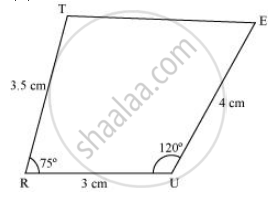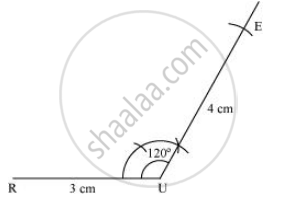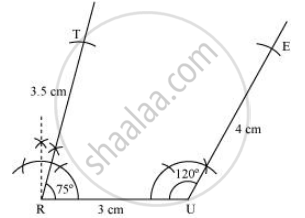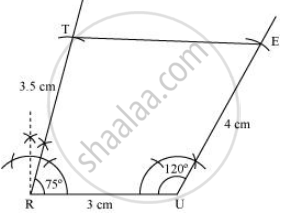# Construct the Following Quadrilaterals, Quadrilateral True Tr = 3.5 Cm Ru = 3 Cm Ue = 4 Cm ∠R = 75° ∠U = 120° - Mathematics

TR = 3.5 cm

RU = 3 cm

UE = 4 cm

∠R = 75°

∠U = 120°

#### Solution

1) A rough sketch of this quadrilateral can be drawn as follows.2) Draw a line segment RU of 3 cm and an angle of 120º at point U. As vertex E is 4 cm away from vertex U, cut a line segment UE of 4 cm
from this ray.3) Next, draw an angle of 75º at point R. As vertex T is 3.5 cm away from vertex R, cut a line segment RT of 3.5 cm from this ray.4) Join T to E.Is there an error in this question or solution?

#### APPEARS IN

NCERT Solution for Mathematics Textbook for Class 8 (2018 (Latest))
Chapter 4: Practical Geometry
Exercise 4.4 | 1.2 | Page no. 67

#### Video TutorialsVIEW ALL 

Construct the Following Quadrilaterals, Quadrilateral True Tr = 3.5 Cm Ru = 3 Cm Ue = 4 Cm ∠R = 75° ∠U = 120° Concept: Constructing a Quadrilateral When Three Sides and Two Included Angles Are Given.